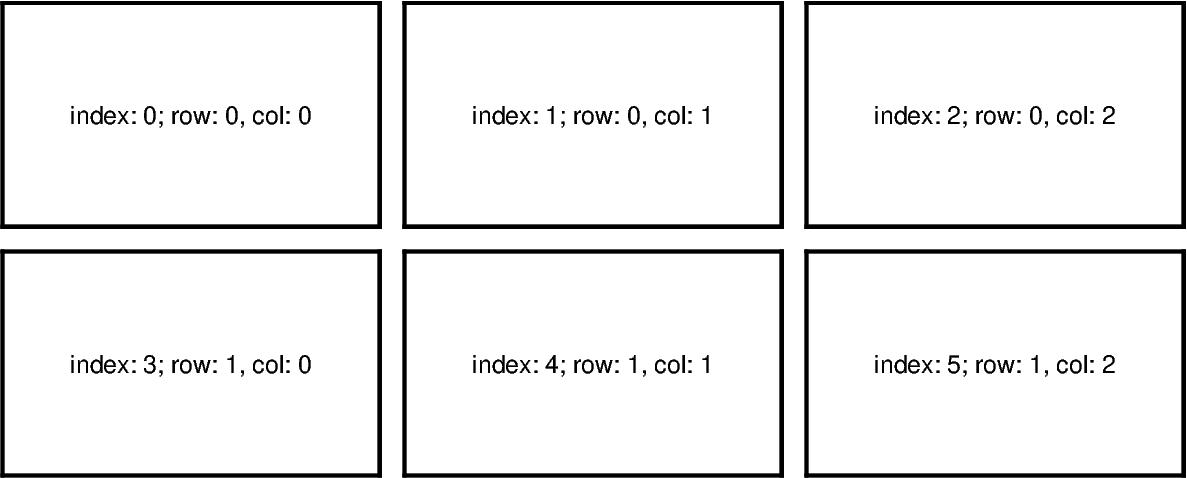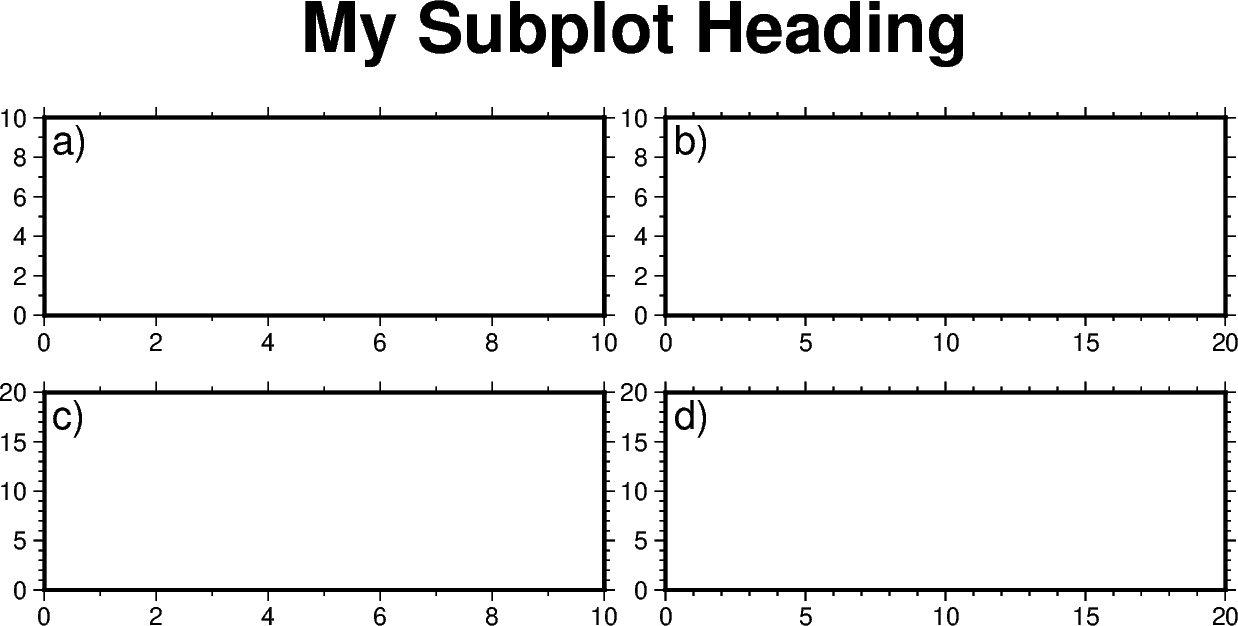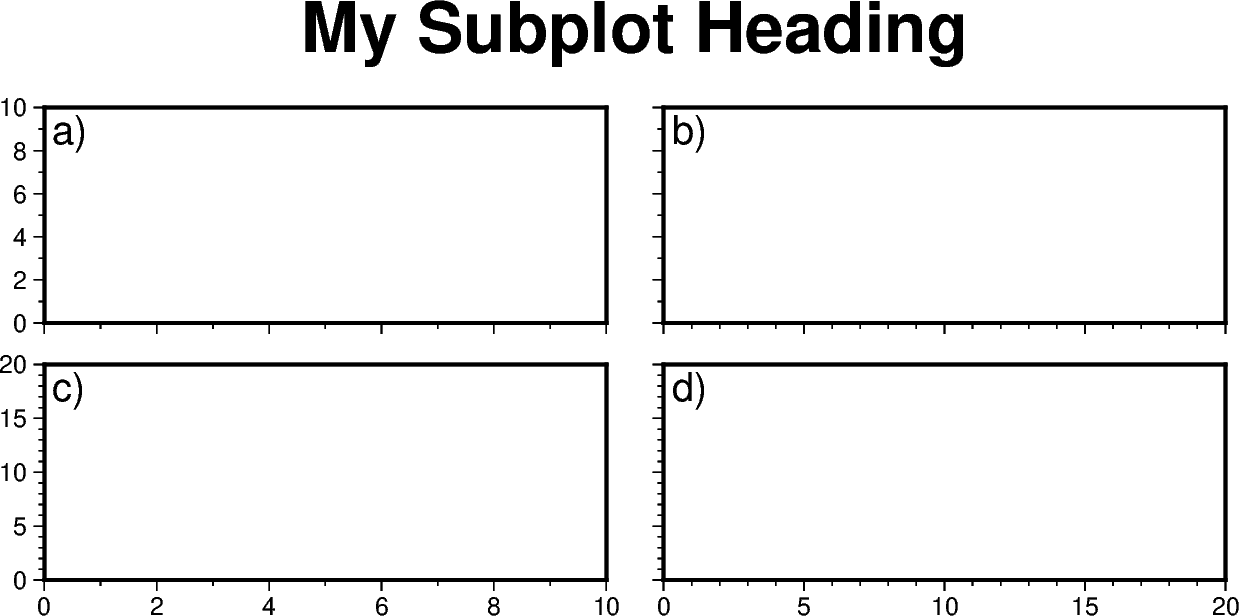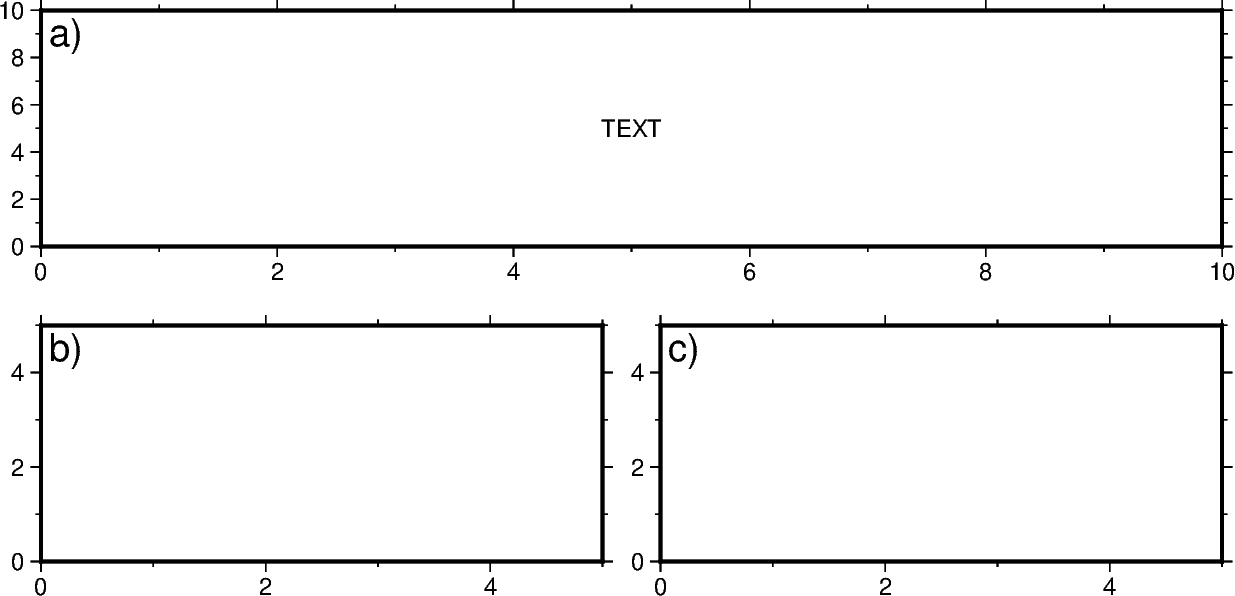# Making subplots¶

When you’re preparing a figure for a paper, there will often be times when you’ll need to put many individual plots into one large figure, and label them ‘abcd’. These individual plots are called subplots.

There are two main ways to create subplots in GMT:

The first method is easier to use and should handle simple cases involving a couple of subplots. For more advanced subplot layouts, however, we recommend the use of `pygmt.Figure.subplot` which offers finer grained control, and this is what the tutorial below will cover.

```import pygmt
```

Let’s start by initializing a `pygmt.Figure` instance.

```fig = pygmt.Figure()
```

## Define subplot layout¶

The `pygmt.Figure.subplot` function is used to set up the layout, size, and other attributes of the figure. It divides the whole canvas into regular grid areas with n rows and m columns. Each grid area can contain an individual subplot. For example:

```with fig.subplot(nrows=2, ncols=3, figsize=("15c", "6c"), frame="lrtb"):
...
```

will define our figure to have a 2 row and 3 column grid layout. `figsize=("15c", "6c")` defines the overall size of the figure to be 15 cm wide by 6 cm high. Using `frame="lrtb"` allows us to customize the map frame for all subplots instead of setting them individually. The figure layout will look like the following:

```with fig.subplot(nrows=2, ncols=3, figsize=("15c", "6c"), frame="lrtb"):
for i in range(2):  # row number starting from 0
for j in range(3):  # column number starting from 0
index = i * 3 + j  # index number starting from 0
with fig.set_panel(panel=index):  # sets the current panel
fig.text(
position="MC",
text=f"index: {index}; row: {i}, col: {j}",
region=[0, 1, 0, 1],
)
fig.show()
```Out:

```<IPython.core.display.Image object>
```

The `pygmt.Figure.set_panel` function activates a specified subplot, and all subsequent plotting functions will take place in that subplot panel. This is similar to matplotlib’s `plt.sca` method. In order to specify a subplot, you will need to provide the identifier for that subplot via the `panel` parameter. Pass in either the index number, or a tuple/list like (row, col) to `panel`.

Note

The row and column numbering starts from 0. So for a subplot layout with N rows and M columns, row numbers will go from 0 to N-1, and column numbers will go from 0 to M-1.

For example, to activate the subplot on the top right corner (index: 2) at row=0 and col=2, so that all subsequent plotting commands happen there, you can use the following command:

```with fig.set_panel(panel=[0, 2]):
...
```

Next, let’s use what we learned above to make a 2 row by 2 column subplot figure. We’ll also pick up on some new parameters to configure our subplot.

```fig = pygmt.Figure()
with fig.subplot(
nrows=2,
ncols=2,
figsize=("15c", "6c"),
autolabel=True,
frame=["af", "WSne"],
margins=["0.1c", "0.2c"],
):
fig.basemap(region=[0, 10, 0, 10], projection="X?", panel=[0, 0])
fig.basemap(region=[0, 20, 0, 10], projection="X?", panel=[0, 1])
fig.basemap(region=[0, 10, 0, 20], projection="X?", panel=[1, 0])
fig.basemap(region=[0, 20, 0, 20], projection="X?", panel=[1, 1])
fig.show()
```Out:

```<IPython.core.display.Image object>
```

In this example, we define a 2-row, 2-column (2x2) subplot layout using `pygmt.Figure.subplot`. The overall figure dimensions is set to be 15 cm wide and 6 cm high (`figsize=["15c", "6c"]`). In addition, we use some optional parameters to fine-tune some details of the figure creation:

• `autolabel=True`: Each subplot is automatically labelled abcd

• `margins=["0.1c", "0.2c"]`: adjusts the space between adjacent subplots. In this case, it is set as 0.1 cm in the X direction and 0.2 cm in the Y direction.

• `title="My Subplot Heading"`: adds a title on top of the whole figure.

Notice that each subplot was set to use a linear projection `"X?"`. Usually, we need to specify the width and height of the map frame, but it is also possible to use a question mark `"?"` to let GMT decide automatically on what is the most appropriate width/height for the each subplot’s map frame.

Tip

In the above example, we used the following commands to activate the four subplots explicitly one after another:

```fig.basemap(..., panel=[0, 0])
fig.basemap(..., panel=[0, 1])
fig.basemap(..., panel=[1, 0])
fig.basemap(..., panel=[1, 1])
```

In fact, we can just use `fig.basemap(..., panel=True)` without specifying any subplot index number, and GMT will automatically activate the next subplot panel.

Note

All plotting functions (e.g. `pygmt.Figure.coast`, `pygmt.Figure.text`, etc) are able to use `panel` parameter when in subplot mode. Once a panel is activated using `panel` or `pygmt.Figure.set_panel`, subsequent plotting commands that don’t set a `panel` will have their elements added to the same panel as before.

## Shared X and Y axis labels¶

In the example above with the four subplots, the two subplots for each row have the same Y-axis range, and the two subplots for each column have the same X-axis range. You can use the `sharex`/`sharey` parameters to set a common X and/or Y axis between subplots.

```fig = pygmt.Figure()
with fig.subplot(
nrows=2,
ncols=2,
figsize=("15c", "6c"),  # width of 15 cm, height of 6 cm
autolabel=True,
margins=["0.3c", "0.2c"],  # horizontal 0.3 cm and vertical 0.2 cm margins
sharex="b",  # shared x-axis on the bottom side
sharey="l",  # shared y-axis on the left side
frame="WSrt",
):
fig.basemap(region=[0, 10, 0, 10], projection="X?", panel=True)
fig.basemap(region=[0, 20, 0, 10], projection="X?", panel=True)
fig.basemap(region=[0, 10, 0, 20], projection="X?", panel=True)
fig.basemap(region=[0, 20, 0, 20], projection="X?", panel=True)
fig.show()
```Out:

```<IPython.core.display.Image object>
```

`sharex="b"` indicates that subplots in a column will share the x-axis, and only the bottom axis is displayed. `sharey="l"` indicates that subplots within a row will share the y-axis, and only the left axis is displayed.

Of course, instead of using the `sharex`/`sharey` option, you can also set a different `frame` for each subplot to control the axis properties individually for each subplot.

Nested subplot are currently not supported. If you want to create more complex subplot layouts, some manual adjustments are needed.

The following example draws three subplots in a 2-row, 2-column layout, with the first subplot occupying the first row.

```fig = pygmt.Figure()
# Bottom row, two subplots
with fig.subplot(nrows=1, ncols=2, figsize=("15c", "3c"), autolabel="b)"):
fig.basemap(
region=[0, 5, 0, 5], projection="X?", frame=["af", "WSne"], panel=[0, 0]
)
fig.basemap(
region=[0, 5, 0, 5], projection="X?", frame=["af", "WSne"], panel=[0, 1]
)
# Move plot origin by 1 cm above the height of the entire figure
fig.shift_origin(yshift="h+1c")
# Top row, one subplot
with fig.subplot(nrows=1, ncols=1, figsize=("15c", "3c"), autolabel="a)"):
fig.basemap(
region=[0, 10, 0, 10], projection="X?", frame=["af", "WSne"], panel=[0, 0]
)
fig.text(text="TEXT", x=5, y=5)

fig.show()
```Out:

```<IPython.core.display.Image object>
```

We start by drawing the bottom two subplots, setting `autolabel="b)"` so that the subplots are labelled ‘b)’ and ‘c)’. Next, we use `pygmt.Figure.shift_origin` to move the plot origin 1 cm above the height of the entire figure that is currently plotted (i.e. the bottom row subplots). A single subplot is then plotted on the top row. You may need to adjust the `yshift` parameter to make your plot look nice. This top row uses `autolabel="a)"`, and we also plotted some text inside. Note that `projection="X?"` was used to let GMT automatically determine the size of the subplot according to the size of the subplot area.

You can also manually override the `autolabel` for each subplot using for example, `fig.set_panel(..., fixedlabel="b) Panel 2")` which would allow you to manually label a single subplot as you wish. This can be useful for adding a more descriptive subtitle to individual subplots.

Total running time of the script: ( 0 minutes 6.382 seconds)

Gallery generated by Sphinx-Gallery